Quantity: 0

Total: 0,00

0

# Cuboid

### Cuboid

A cuboid is a polyhedron with six rectangular faces.

Mathematics

Keywords

cuboid, vertex, edge, face, face diagonal, space diagonal

Related items

### Related items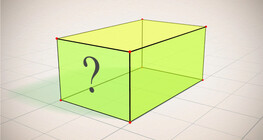#### Cuboid (exercises)

Edges, diagonals and faces of a cuboid can be identified by its vertices.#### Grouping of cuboids

This animation demonstrates various types of cuboids through everyday objects.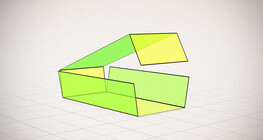#### Nets of a cuboid (exercises)

This animation demonstrates the different nets of a cuboid and includes a game.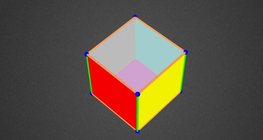#### Colouring a cube

Colouring the vertices, edges and faces of a given cube according to the criteria specified in the exercise.#### Cube

This animation demonstrates the components (vertices, edges, diagonals and faces) of the cube, one of the Platonic solids.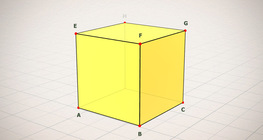#### Cube (exercises)

Edges, diagonals and faces of a cube can be identified by its vertices.#### Cube of cubes

An exercise about the regular hexahedron built from unit cubes to help deepen your knowledge of cubes.#### Cube puzzle

Building cubes shown in several views from the available unit cubes aids spatial vision and other skills.#### Cube sections (exercise)

Examining solids formed by the intersection of a cube and a plane.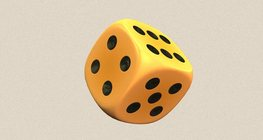#### Dice

Regular dice can be used for solving statistical and probability exercises.#### Grouping of solids 1

This animation demonstrates various groups of solids through examples.#### Net of a cube (exercises)

Not all nets consisting of 6 congruent squares are foldable into cubes.#### Perimeter, area, surface area and volume

This animation presents the formulas to calculate the perimeter and area of shapes as well as the surface area and volume of solids.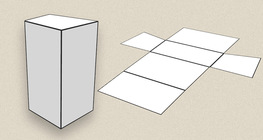#### Prisms

This animation demonstrates several types of prisms, from general to regular.#### Ratio of volumes of similar solids

This 3D scene explains the correlation between the ratio of similarity and the ratio of volume of geometric solids.#### Volume and surface area (exercise)

An exercise about the volume and surface area of solids generated from a ´base cube´.#### Dividing Space into Regions by 3 Planes

Division of space by three intersecting planes in several different arrangements.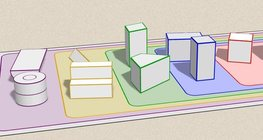#### Grouping of solids

This animation demonstrates various groups of solids through examples.